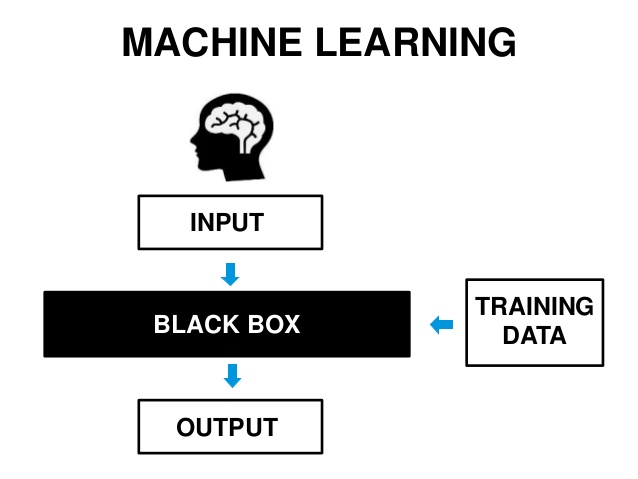Wanna know more about data science? Make sure to check out my events and my webinar What it's like to be a data scientist and What’s the best way to become a data scientist !

# Solving machine learning problems

Solving a machine learning problem can be a daunting affair for beginner data scientists. There are simply so many algorithms to choose from! Simply go to scikit-learn‘s page and you are already overwhelmed by all the options! One of the main challenges is that if you get bad performance results, you can’t be sure whether it is your fault, or the dataset is simply not good enough.

Through all the years of practice, I have developed a process which I am using to quickly figure out whether the data is of good quality or not.

In machine learning algorithms can be placed on a continuum of “power”, from the least powerful to the most powerful ones. Naive Bayes for example, is a very simply classifier. Deep neural networks and random forests, on the other hand, are very powerful models. In terms of regression, linear regression is probably the simplest regression algorithm in existence.

Now, let’s see how we can use this, in order to quickly figure out whether there is something wrong with the dataset or something wrong with our approach.# A fast process for machine learning problems

So, the trick is simply as follows:

1. Use 1-2 very simple models. Record the results.
2. Use 1-2 very complicated models. Record the results.

If the results are very similar, then this means that it is difficult for the more powerful models to extract more information from the dataset than the simple models. So, what this means is that it is very likely that there is simply not enough useful information in the dataset.

So, for example, let’s say that you used linear regression for a regression task, and you are getting an RMSE of 2.5. And then you are using a random forest with a large number of trees, e.g. 500 trees, for a dataset of 50 features. If the performance (in terms of RMSE) is something like 2.34, then this means that the random forest finds it difficult to extract more information than a simple linear model.# How to use this process for machine learning problems

The law of parsimony states that you want to use the simplest possible model that works well for a given problem. So, what you want to do, is you want to make sure that you are not using more complicated models than needed.

With this simple process I outlined you can make sure that do exactly that.

Obviously, if you want to be more thorough about a problem, there are many more steps that you will have to take:

1. Re-examine the quality of the data.
2. Understand whether you can collect more data.
3. Think of potential features which can be extracted from the dataset, in order to further improve performance.

That being said, however, if you keep seeing that simple models have very similar performance to complicated ones, then you can be sure that simply adding even more complexity into the mix, it is unlikely to benefit you much. Good data with average algorithms, will usually overperform bad data with excellent algorithms. So, if you are a beginner in data science, make sure to focus on approaching problems holistically, instead of simply trying models until you find something that works.

Wanna know more about data science? Besides my events, you should check out my webinars:
1. If you want to learn data science: What it's like to be a data scientist and What’s the best way to become a data scientist
2. If you are a CEO: The importance of data strategy

Dr. Stylianos Kampakis is the owner and author of The Data Scientist.